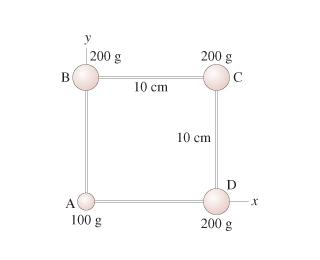# find the moment of inertia about a diagonal axis that passes through masses b and d.

edited

find the moment of inertia about a diagonal axis that passes through masses b and d.

The four masses shown in the figure are connected by massless, rigid rods.Find the moment of inertia about a diagonal axis that passes through masses B and D.

x-coordinate xcm=5.7 cm; y-coordinate ycm=5.7cm

by

I = m*r^2 for point masses. So you need the distance of points A & C from the diagonal which is 7.07cm. So I = (.100kg)*(.0707m)^2 + (.200kg)*(.0707m)^2 = 0.00150 kg-m^2

Lorem ipsum dolor sit amet, consectetur adipiscing elit, sed do eiusmod tempor incididunt ut labore et dolore magna aliqua. Ut enim ad minim veniam, quis nostrud exercitation ullamco laboris nisi ut aliquip ex ea commodo consequat. Duis aute irure dolor in reprehenderit in voluptate velit esse cillum dolore eu fugiat.

+1 vote